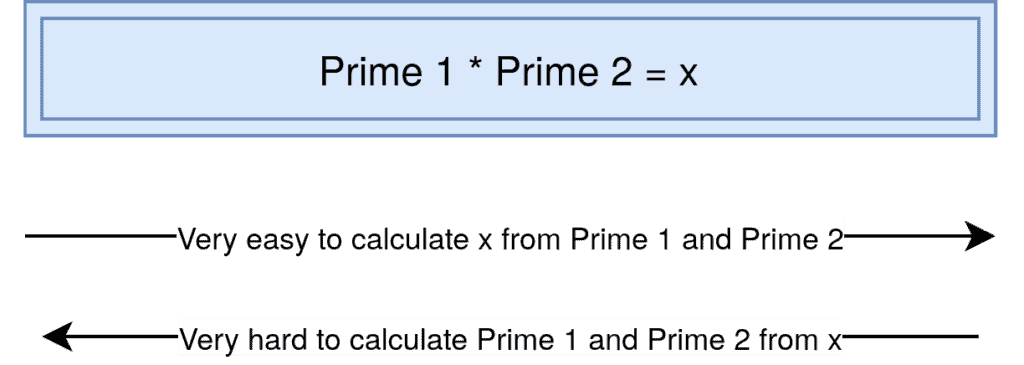## 1. Introduction

In this tutorial, we’re going to explore why prime numbers are important in cryptography. We do this by looking at a specific cryptosystem, namely the RSA algorithm. While the methods used in the application of the RSA algorithm contain lots of details to keep the encryption as secure as possible, we’ll focus on the main aspects of it.

## 2. The Special Property of Prime Numbers

Every number can be factorized into its prime numbers. Generally, it’s very hard to find the factors of a number. To find all the prime factors of a natural number, one has to try and divide it by its possible factors up to.

It is very difficult to find the prime factors of a large number. On the other hand, it’s very easy to calculate a number with already given primes:Ideally, we use two big numbers which are primes. We then calculate the product of those two to encrypt a message. To decrypt it we need one of the primes because there’s no easy way to calculate Prime 1 and Prime 2 fromalone. But before we go into the details of how we can use these numbers exactly, let’s take a look at the different cryptographic systems.

## 3. Cryptographic Systems

In cryptography, we have two important methods to encrypt messages: symmetric encryption and asymmetric encryption.

In the symmetric case, both parties share the same key. We use the same key to encrypt and decrypt a message. It’s very safe as long as only the two people have the key and they have a way to share it with each other safely, for example in person.

Now we can imagine that this method is very hard to implement. If we want to write an encrypted E-Mail to someone we shouldn’t first have to meet in person with him to exchange the secret keys.

That’s why, in asymmetric encryption, we have two different keys, one for encrypting and one for decrypting. One key is for the writer of the message. After writing his message he can encrypt it with the public key from the recipient. This key is, as the name suggests, public, and can be looked up i.e. in a key database. As it’s only for encrypting it does no harm by being public. On the other hand, there’s the private key. This key is only visible to one person, the recipient of the messages. He can use it to decrypt the messages he receives:## 4. Using Prime Numbers for Encryption

Now that we have a clear understanding of the two different encryption systems, let’s take a look at how we can create a public and a private key in the case of asymmetric encryption.

First, we should note that we can not encrypt the text directly, but have to transform it to a number first. This process is called padding and happens with a list that assigns a number to each symbol. Then we connect each number to create another number, let’s call it, which we then encrypt. A very easy padding list is just assigning each letter to its position in the alphabet, for example, “A” to 1, “B” to 2, etc. While this list only allows very simple words it’s sufficient to understand the theory behind RSA.

### 4.1. Creating a Key

As we have already mentioned in the second paragraph, it’s very easy to calculate a large number from known primes. On the other hand, it’s very hard to guess the factors of a known large number. We use this mechanism in the following process, to create two keys, a private and a public one:

1. Choose two random, stochastically independent prime numbers,and2. Calculate the product of both3. Calculate the Phi function of both:4. Choose a natural numberthat’s coprime to and smaller than5. Calculate the multiplicative inverseofmodulo, i.e.andnow build our public keys, we’ll use them to encrypt the message. Our Inverse, which we use to decrypt the encrypted message,on the other hand is our private key. To see this more clearly we have a look at the encryption and decryption process.

### 4.2. Encrypting and Decrypting a Message

We now encrypt our messageby using the public key:and we decrypt it with:As we can see we can only invert our encryption if we have the multiplicative inverseofmod. These we can only get if we have either

• The private key
• The prime factors ofSince it’s not possible to calculate the prime factors of a largein the foreseeable future, there’s no way to decrypt the message without the private key. This makes the system very secure.

## 5. An Example

To get a feeling of how the algorithm works let’s calculate an example.

### 5.1. Creating the Key

The letter that we want to encrypt is “O” and we transform it into the numbersince it’s the fifteenth number of the alphabet. Now we choose our random primes. To make things simple we choose the primesand.

Then we build the phi function of the primes withWe also choose a numberthat’s coprime to, let’s take 29.

The only thing left to calculate is the inverseof. With help of the euclidean algorithm, we calculate it to be 53.

Thus we have the public key. And also our private key.

### 5.2. Encryption and Decryption

Next we encrypt our Number:This yieldsWe now have our encrypted message and can safely transfer it to the recipient without anyone being able to know that it stands for the letter “O”.

To decrypt the message we need our inversewhich is 53:Now we look again at the alphabet and see that the fifteenth letter is “O”, meaning we have successfully encrypted and decrypted our message.

## 6. Conclusion

As we have seen, we can use the inability to factor large numbers into its primes to generate a safe, asymmetric cryptographic system.